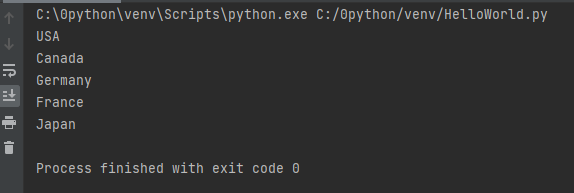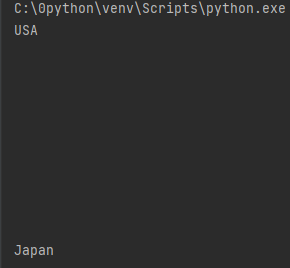# Python Print() 语句：通过示例介绍打印语句

## Python print() 函数

Python 中的 print() 函数用于在屏幕上打印指定的消息。 Python 中的 print 命令打印在屏幕上打印出字符串或转换为字符串的对象。

``print(object(s))``

## 如何在 Python 中打印一个简单的字符串？

``print ("welcome to 2743")``

``welcome to 2743``

``````print("USA")
print("Germany")
print("France")
print("Japan")``````## 如何打印空行

``print (8 * "\n")``

``print ("\n\n\n\n\n\n\n\n\n")``

``````print("USA")
print (8 * "\n")
print("Japan")``````## 打印结束命令

``````print ("Welcome to", end = ' ')
print ("2743", end = '!')``````

``Welcome to 2743!``

# ends the output with ‘@.’

``print("Python" , end = '@')``

``Python@``

(Visited 14 times, 1 visits today)

Posted in Python教程